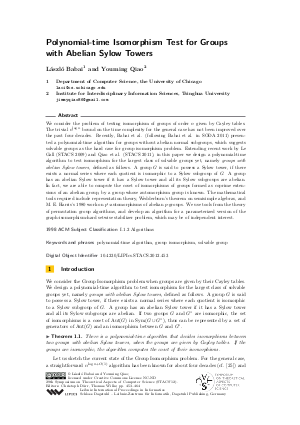Document# Polynomial-time Isomorphism Test for Groups with Abelian Sylow Towers

### Authors László Babai, Youming Qiao## File

LIPIcs.STACS.2012.453.pdf
• Filesize: 0.59 MB
• 12 pages

## Cite As

László Babai and Youming Qiao. Polynomial-time Isomorphism Test for Groups with Abelian Sylow Towers. In 29th International Symposium on Theoretical Aspects of Computer Science (STACS 2012). Leibniz International Proceedings in Informatics (LIPIcs), Volume 14, pp. 453-464, Schloss Dagstuhl - Leibniz-Zentrum für Informatik (2012)
https://doi.org/10.4230/LIPIcs.STACS.2012.453

## Abstract

We consider the problem of testing isomorphism of groups of order n given by Cayley tables. The trivial n^{log n} bound on the time complexity for the general case has not been improved over the past four decades. Recently, Babai et al. (following Babai et al. in SODA 2011) presented a polynomial-time algorithm for groups without abelian normal subgroups, which suggests solvable groups as the hard case for group isomorphism problem. Extending recent work by Le Gall (STACS 2009) and Qiao et al. (STACS 2011), in this paper we design a polynomial-time algorithm to test isomorphism for the largest class of solvable groups yet, namely groups with abelian Sylow towers, defined as follows. A group G is said to possess a Sylow tower, if there exists a normal series where each quotient is isomorphic to Sylow subgroup of G. A group has an abelian Sylow tower if it has a Sylow tower and all its Sylow subgroups are abelian. In fact, we are able to compute the coset of isomorphisms of groups formed as coprime extensions of an abelian group, by a group whose automorphism group is known. The mathematical tools required include representation theory, Wedderburn's theorem on semisimple algebras, and M.E. Harris's 1980 work on p'-automorphisms of abelian p-groups. We use tools from the theory of permutation group algorithms, and develop an algorithm for a parameterized versin of the graph-isomorphism-hard setwise stabilizer problem, which may be of independent interest.
##### Keywords
• polynomial-time algorithm
• group isomorphism
• solvable group

## Metrics

• Access Statistics
• Total Accesses (updated on a weekly basis)
0